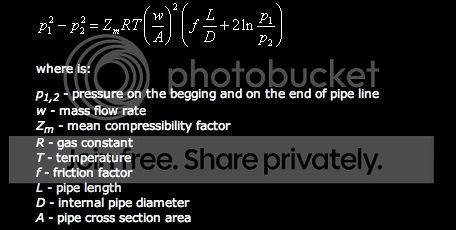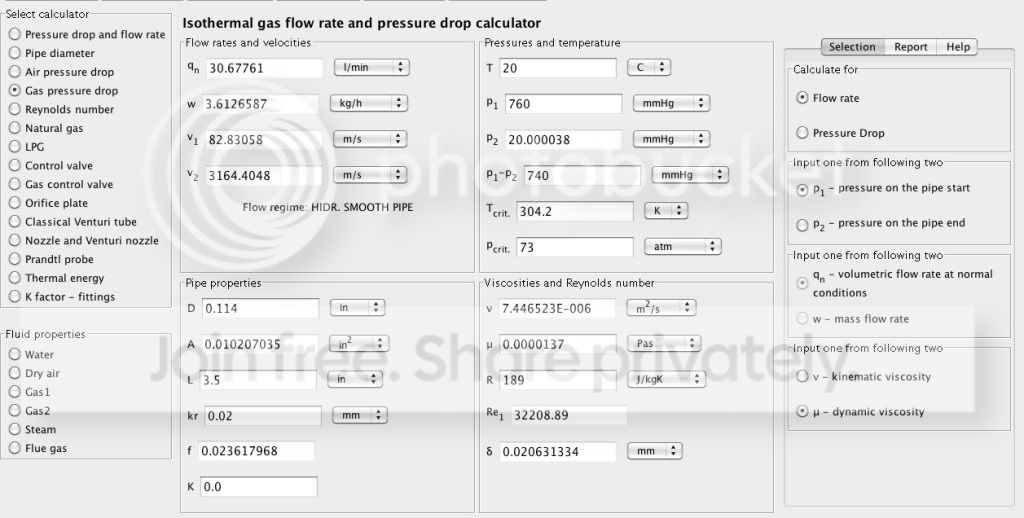## Gas Pressure Drop Theory Check

Forum about flow calculators available on pipeflowcalculations.com and how to find solution for your fluid flow problem.
lakid594
Posts: 2
Joined: Tue Jul 19, 2011 6:15 pm

### Gas Pressure Drop Theory Check

what are the correct units to use in each of the variables in the adiabatic compressible flow equation for the gas pressure drop?

the equation is here:
http://www.pipeflowcalculations.com/pip ... l-flow.phpthis is the result i received from the calculator:I am trying to calculate the flow in liters/min of CO2 through a pipe with D=.114 in and L=3.5 in, p1=760 mmHg, p2= 20mmHg. I used the gas pressure drop calculator, but I want to check my work by hand because as the pressure difference increases, the flow rate decreases (when P1-P2 is larger than about 290 mmHg). Am I using the right equation to do so? if so, what units should i be using with each of the variables?